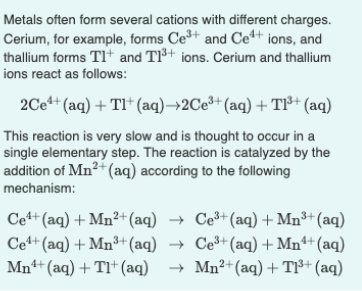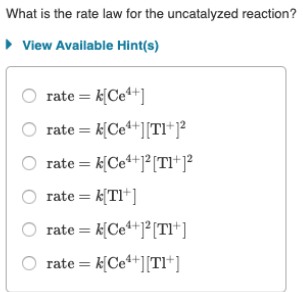# Metals often form several cations with different charges. Cerium, for example, forms Ce^3+ and Ce^4+ ions, and thallium forms Tl^+ and Tl^3+ ions. Cerium and thallium ions react as follows: 2Ce^4+ (aq) + Tl^+ (aq) → 2Ce^3+(aq) + Tl^3+ (aq) . This reaction is very slow and is thought to occur in a single elementary step. The reaction is catalyzed by the addition of Mn^2+ (aq) according to the following mechanism: Ce^4+ (aq) + Mn^2+(aq) → Ce^3+(aq) + Mn^3+(aq) Ce^4+ (aq) + Mn^3+(aq) → Ce^3+(aq) + Mn^4+(aq) Mn^4+(aq) + Tl^+(aq) → Mn^2+(aq) + Tl^3+(aq) What is the rate law for the uncatalyzed reaction? a) rate = k[Ce^4+] b) rate = k[Ce^4+] [Tl^+]^2 c) rate = k[Ce^4+]^2 [Tl^+]^2 d) rate = k[Tl^+] e) rate = k[Ce^4+]^2 [Tl^+] f) rate = k[Ce^4+] [Tl^+]